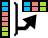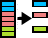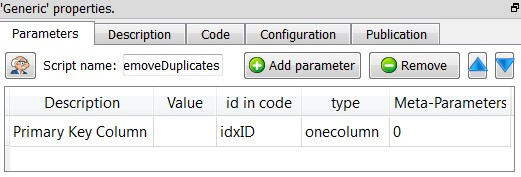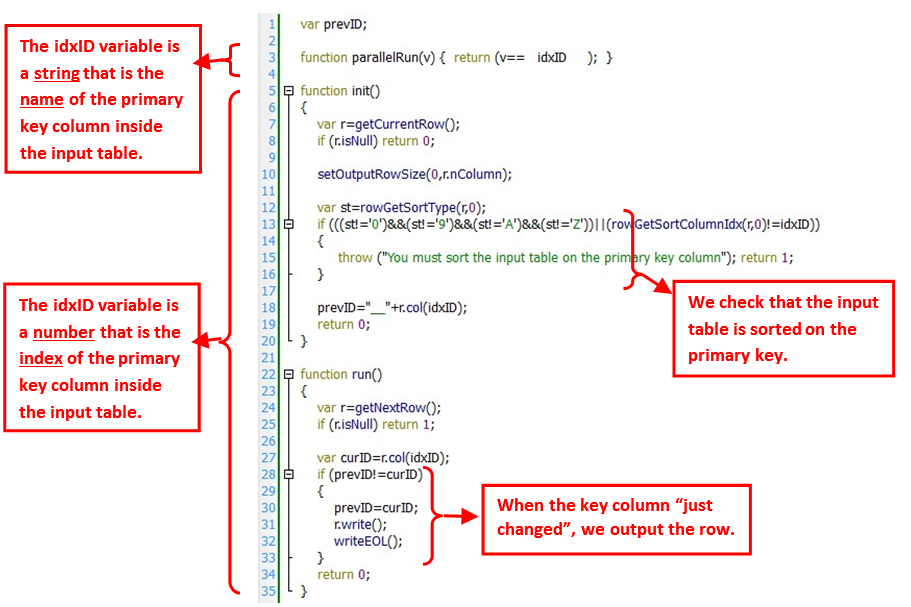﻿ 9. Anatella for the Expert Users > 9.9. Executing Scripts inside a N-Way Multithread section

# 9.9. Executing Scripts inside a N-Way Multithread section

Navigation:  9. Anatella for the Expert Users >

# 9.9. Executing Scripts inside a N-Way Multithread section

Not all Actions can be included inside a N-Way section.

# 9.9.1. For JavaScript-based Actions

Some Action can be included inside a N-Way section only if the partitioning variable of the N-Way section is a specific variable. For example: the“Flatten” Action can be included inside a N-Way section only of the partitioning variable of the N-Way section is equal to the “Key Column” parameter of the“Flatten” Action.

Let’s take an example: Let’s write in JavaScript a “simple Row De-Duplicate” (more or less equivalent to theNaïveDeDuplicate Action). There is only one parameter to the SimplifiedRemoveDuplicate Action: Which column is the primary key?The JavaScript code is:The algorithm of the “SimplifiedRemoveDuplicate Action” is very simple: We check that the input table is sorted on the key column. Because of the sort, all the rows with the same key-column are “grouped together” in contiguous rows. We read the (sorted) input table row-by-row. When we receive a row with a different key-column as the previous row (i.e. when the key column “just changed”), we output the row.

The above algorithm works inside a N-Way Multithreaded Section only if the partitioning variable of the N-Way section is equal to the “Primary Key” parameter of the “SimplifiedRemoveDuplicate Action” (i.e. is equal to the idxID variable). This check is performed inside the “parallelRun(v)” function. The content of the argument “v” to the “parallelRun(v)” function is the name of the partitioning variable of the N-Way section. The content of the variable “idxID” is slightly different inside the the “parallelRun(v)” function than inside the other functions (i.e. inside “init()” and “run()”): it contains the name of the selected column (instead of the index of the selected column).

# 9.9.2. For R/Python based Actions

A given R or Python Action can work inside a N-Way Multithreaded Section only if the “Partition Type” is:

Each Partition has the same number of rows (with the exception of the last partition)

Partition by Column: When using this option, you must select a “Partitioning Column” that is equal to the partitioning variable of the N-Way section.

When the “Partition Type” is “No partition” (this is the default option), then the R/Python Action won’t work inside a N-Way Multithreaded Section.